Home | ARTS | Operations Management | Method of convex linear combination - The Principle Of Dominance

# Method of convex linear combination - The Principle Of Dominance

Posted On :  24.06.2018 11:55 pm

A strategy, say s, can also be dominated if it is inferior to a convex linear combination of several other pure strategies.

Method of convex linear combination

A strategy, say s, can also be dominated if it is inferior to a convex linear combination of several other pure strategies. In this case if the domination is strict, then the strategy s can be deleted. If strategy s dominates the convex linear combination of some other pure strategies, then one of the pure strategies involved in the combination may be deleted. The domination will be decided as per the above rules. Let us consider an example to illustrate this case.

Problem 2

Solve the game with the following pay-off matrix for firm A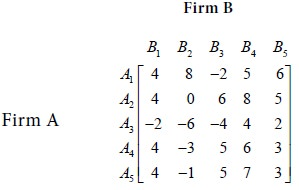Solution

First consider the minimum of each rowMaximum of {-2, 0, -6, -3, -1} = 0

Next consider the maximum of each column.Minimum of { 4, 8, 6, 8, 6}= 4

Hence,

Maximum of {row minima}   minimum of {column maxima}

So we see that there is no saddle point. Compare the second row with the fifth row. Each element in the second row exceeds the corresponding element in the fifth row. Therefore, A2 dominates A5 . The choice is for firm A. It will retain strategy A2 and give up strategy A5 . Therefore the game reduces to the following.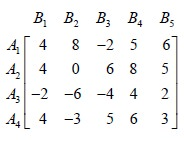Compare the second and fourth rows. We see that A2 dominates A4 . So, firm A will retain the strategy A2 and give up the strategy A4 . Thus the game reduces to the following: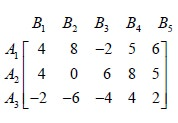Compare the first and fifth columns. It is observed that B1 dominates B5. The choice is for firm B. It will retain the strategy B1 and give up the strategy B5 . Thus the game reduces to the following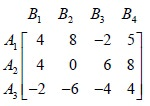Compare the first and fourth columns. We notice that B1 dominates B4. So firm B will discard the strategy B4 and retain the strategy B1 . Thus the game reduces to the following:For this reduced game, we check that there is no saddle point.

Now none of the pure strategies of firms A and B is inferior to any of their other strategies. But, we observe that convex linear combination of the strategies B2 and B3 dominates B1 , i.e. the averages of payoffs due to strategies B2 and B3 ,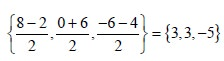dominate B1 . Thus B1 may be omitted from consideration. So we have the reduced matrix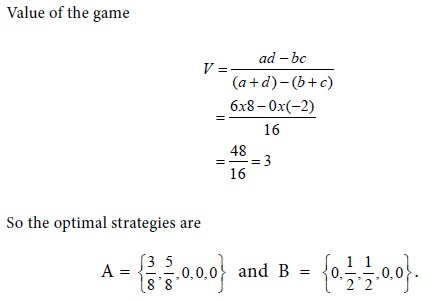The value of the game = 3. Thus the game is favourable to firm A.

Problem 3

For the game with the following pay-off matrix, determine the saddle point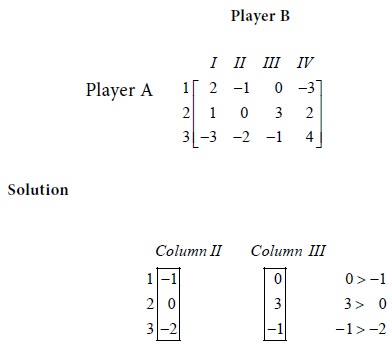The choice is with the player B. He has to choose between strategies II  and III. He will lose more in strategy III than in strategy II, irrespective of what strategy is followed by A. So he will drop strategy III and retain strategy II. Now the given game reduces to the following game.Maximum of row minimum = Minimum of column maximum = 0.

So, a saddle point exists for the given game and the value of the game is 0.

Interpretation

No player gains and no player loses. i.e., The game is not favourable to any player. i.e. It is a fair game.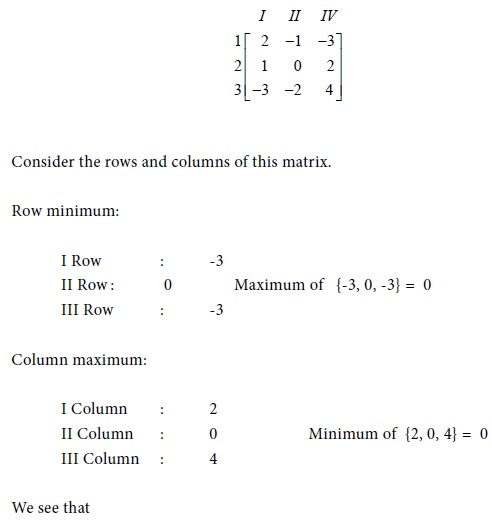Since Maximum of { Row Minima} and Minimum of { Column Maxima } are different, it follows that the given game has no saddle point.

Denote the strategies of player A by A1 , A2 , A3 . Denote the strategies of player B by B1 , B2 , B3 . Compare the first and third columns of the given matrix.The pay-offs in B3 are greater than or equal to the corresponding pay-offs in B1 . The player B has to make a choice between his strategies 1 and 3. He will lose more if he follows strategy 3 rather than strategy 1. Therefore he will give up strategy 3 and retain strategy 1. Consequently, the given game is transformed into the following game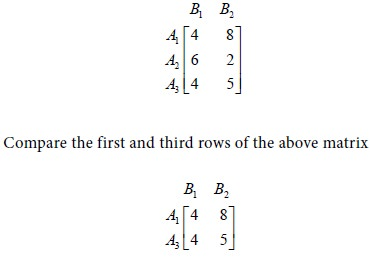The pay-offs in A1 are greater than or equal to the corresponding pay-offs in A3 . The player A has to make a choice between his strategies 1 and 3. He will gain more if he follows strategy 1 rather than strategy 3. Therefore he will retain strategy 1 and give up strategy 3. Now the given game is transformed into the following game.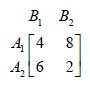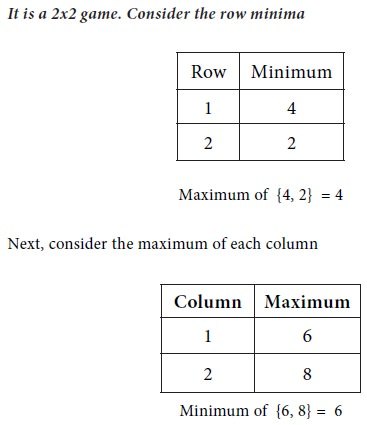Maximum {row minima} and Minimum {column maxima } are not equal Therefore, the reduced game has no saddle point. So, it is a mixed gameThe probability that player A will use his first strategy is p. This is calculated asThe probability that player B will use his first strategy is r. This is calculated as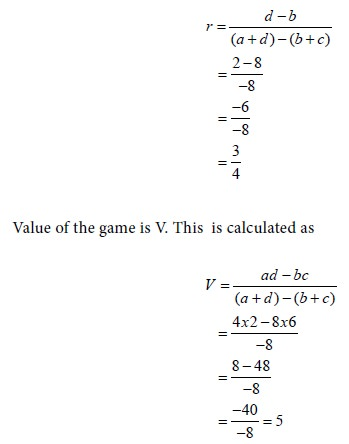Interpretation

Out of 3 trials, player A will use strategy 1 once and strategy 2 once. Out of 4 trials, player B will use strategy 1 thrice and strategy 2 once. The game is favourable to player A.

Problem 5

Dividing a game into sub-games

Solve the game with the following pay-off matrix.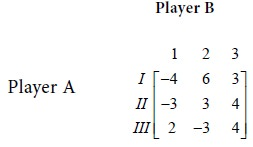We see that Maximum of { row minima} Minimum of { column maxima}.
So the game has no saddle point. Hence it is a mixed game. Compare the first and third columns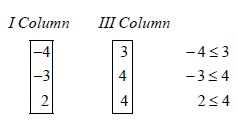We assert that Player B will retain the first strategy and give up the third strategy. We get the following reduced matrix.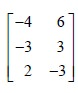We check that it is a game with no saddle point.

Sub games

Let us consider the 2x2 sub games. They are: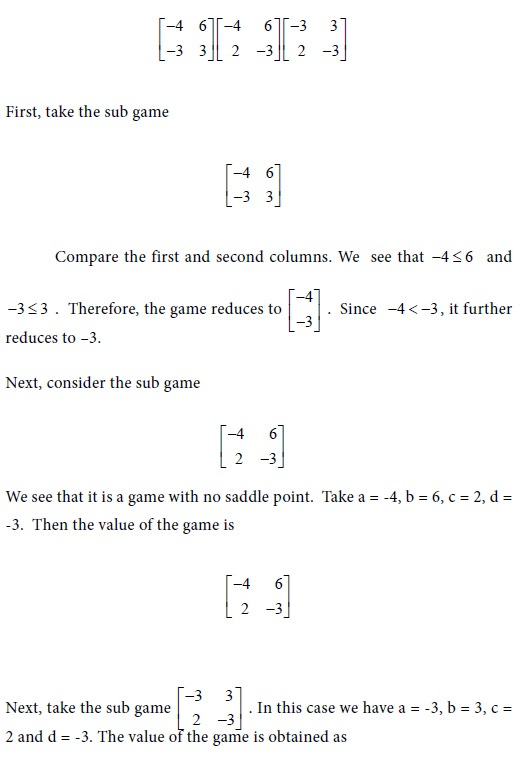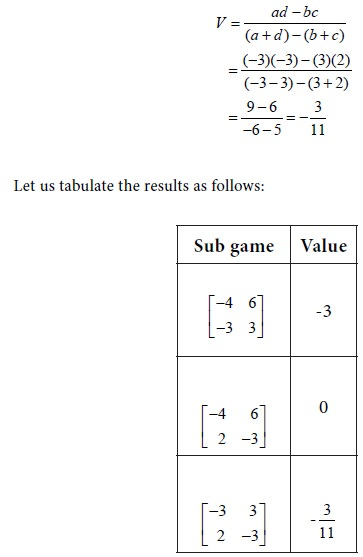The value of 0 will be preferred by the player A. For this value, the first and third strategies of A correspond while the first and second strategies of the player B correspond to the value 0 of the game. So it is a fair game.

Tags : Operations Management - Game Theory, Goal Programming & Queuing Theory
Last 30 days 2322 views